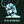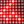0%
0%

# MathSolver

A program written entirely in Python to solve mathematical problems with step-by-step solutions.

Similar projects worth following
233 views
0 followers
As a challenge for my ability to program in Python, I decided to make a program, that I am currently calling "MathSolver", that will display a graphics user interface to the user, where the user can type in any math problem, and the program will compute the step-by-step solution for it. My goal in the end is to make a super intelligent solver that can solve various complex word problems, and this will be useful for anyone who wants to know how to solve them.

In order to create such program from scratch, I have to define the very basics of mathematics, and how their basic operations work. This is similar to how axioms are defined and accepted in mathematics. I will start with defining what a variable, coefficient, and constants are, and how they function with math operations. From there, I will expand onto defining what an expression is, and how to simplify it. I will progress onto solving equations starting with the easiest to the ones that are very complex. And then I will expand onto solving problems of various forms.

As the program becomes more and more advanced, I hope if it is possible to, make the program be able to detect meaning behind word problems. For instance, if the user's word problem asks to find the area, and it gives us the length and width, the program should know that it should multiply the length and width to get the area. Or if it asks us to find the velocity of the ball as soon as it hitss the ground, if it fell off a 100m cliff, then it should be able to use the correct formula, along with some explanations.

The program may take a long time to make, but we'll see how to goes.

• ### Simplifier and Equation Solver Progress

Jasmeet Brar06/27/2016 at 17:04 0 comments

For the last few weeks, I was able to make massive progress in debugging the methods of the variable, constant, and expression classes; and I was able to move forward and make a simplify-by-step method, which would give us the new expression as a result of the simplifying the current expression that we have. Then I moved on and defined a base class for equations in general, and I had defined the one variable equation class, which would inherit from the equation class. The good news about this is that I am able to solve any one variable equations, with some limitations though.

The limitations that I have in my program are:

• It can't handle user input containing parenthesis or brackets in them
• It can't handle variables consisting of more than one letter, where each letter may vary in power
• It can't solve equations that are not first degree.

The biggest limitation is the first one: it can't handle parenthesis and brackets. A lot of problems in mathematics involve these symbols in order to define which operation goes first, or clarify what the inclosed part is. For example, the program must interpret the terms in parenthesis of "x^(2y-5)" as part of the exponent of x.

I could fix this, by tweaking the user input translator so it can handle these operators, but it will be a lot of work, since the meaning of the operator can vary on where it is placed in the expression. It could indicate multiplication, such as 3(5); it could indicate that the containing operations go first, such as 3-(2-5), or it could clarify what the inclosed part is, as mentioned before.

There is a solution to this and that is to implement a data structure known as binary expression tree, as a way to represent expressions. The advantage to this is that it knows which operators goes first, so it doesn't show the parenthesis or brackets in the tree itself. Another advantage is that it allows me to define more functions in the future, without having to go through the mess of handling the brackets. Examples of such functions are: trigonometric functions, and logarithm functions.

This is a massive undertaking, since I would have to take the user input in infix notation, and output a binary tree, or a list of them if it's an equation. I could try to translate the expression in infix notation into Reverse Polish Notation, which may be easier for me to work with. After that, I have to program the simplifier and the one variable equation solver again, so this will set me back. But I think it will be a worthy investment.

The other limitations will be taken care of, after I handle the first one.

I hope I'll be able to make a breakthrough.

• ### Initial Progress

Jasmeet Brar05/01/2016 at 02:35 0 comments

I first started off by creating four files: gui.py, translator.py, definitions.py, and solver.py.

• gui.py is the script that will run the graphics user interface for the user, so that the user can type down their math problem and have it submitted to the program.
• translator.py is the script will perform translations in order to make an expression or an input be in a more friendly form for another function or script to use.
• definitions.py is where I'm going to define all the mathematical objects, and operations, such as: variables, constants, and expressions.
• solver.py is where the logic of solving various problems be stored in.

These four files won't be the only files in this program. I plan to add a script that is dedicated in creating the explanations for the step-by-step solutions, storing each step as it is created, and help project that onto the GUI.

Read more »

Share

## Similar Projects

Project Owner Contributor

### HOM Coin : Efficient Blockchain based real estateDylan Brophy

Project Owner Contributor

### Hackaday Prize Entry: AVATIFYKennyAwesome

# Does this project spark your interest?

Become a member to follow this project and never miss any updates